# Three-way ANOVA in SPSS Statistics

## Introduction

The three-way ANOVA is used to determine if there is an interaction effect between three independent variables on a continuous dependent variable (i.e., if a three-way interaction exists). As such, it extends the two-way ANOVA, which is used to determine if such an interaction exists between just two independent variables (i.e., rather than three independent variables).

Note: It is quite common for the independent variables to be called "factors" or "between-subjects factors", but we will continue to refer to them as independent variables in this guide. Furthermore, it is worth noting that the three-way ANOVA is also referred to more generally as a "factorial ANOVA" or more specifically as a "three-way between-subjects ANOVA".

A three-way ANOVA can be used in a number of situations. For example, you might be interested in the effect of two different types of exercise programme (i.e., type of exercise programme) for improving marathon running performance (i.e., time to run a marathon). However, you are concerned that the effect that each type of exercise programme has on marathon running performance might be different for males and females (i.e., depending on your gender), as well as if you are normal weight or obese (i.e., your body composition). Indeed, you suspect that the effect of the type of exercise programme on marathon running performance will depend on both your gender and body composition. As such, you want to determine if a three-way interaction effect exists between type of exercise programme, gender and body composition (i.e., the three independent variables) in explaining marathon running performance.

In this "quick start" guide, we show you how to carry out a three-way ANOVA using SPSS Statistics, as well as interpret and report the results from this test. However, before we introduce you to this procedure, you need to understand the different assumptions that your data must meet in order for a three-way ANOVA to give you a valid result. We discuss these assumptions next.

## Assumptions

When you choose to analyse your data using a three-way ANOVA, part of the process involves checking to make sure that the data you want to analyse can actually be analysed using a three-way ANOVA. You need to do this because it is only appropriate to use a three-way ANOVA if your data "passes" six assumptions that are required for a three-way ANOVA to give you a valid result. In practice, checking for these six assumptions means that you have a few more procedures to run through in SPSS Statistics when performing your analysis, as well as spend a little bit more time thinking about your data, but it is not a difficult task.

Before we introduce you to these six assumptions, do not be surprised if, when analysing your own data using SPSS Statistics, one or more of these assumptions is violated (i.e., is not met). This is not uncommon when working with real-world data rather than textbook examples, which often only show you how to carry out a three-way ANOVA when everything goes well! However, don’t worry. Even when your data fails certain assumptions, there is often a solution to overcome this. First, let’s take a look at these six assumptions:

• Assumption #1: Your dependent variable should be measured at the continuous level (i.e., it is an interval or ratio variable). Examples of continuous variables include revision time (measured in hours), intelligence (measured using IQ score), exam performance (measured from 0 to 100), weight (measured in kg), and so forth. You can learn more about interval and ratio variables in our article: Types of Variable.
• Assumption #2: Your three independent variables should each consist of two or more categorical, independent groups. Example independent variables that meet this criterion include gender (two groups: male or female), ethnicity (three groups: Caucasian, African American and Hispanic), profession (five groups: surgeon, doctor, nurse, dentist, therapist), and so forth.
• Assumption #3: You should have independence of observations, which means that there is no relationship between the observations in each group or between the groups themselves. For example, there must be different participants in each group with no participant being in more than one group. This is more of a study design issue than something you would test for, but it is an important assumption of the three-way ANOVA. If your study fails this assumption, you will need to use another statistical test instead of the three-way ANOVA (e.g., a repeated measures design). If you are unsure whether your study meets this assumption, you can use our Statistical Test Selector, which is part of our enhanced guides.
• Assumption #4: There should be no significant outliers. Outliers are data points within your data that do not follow the usual pattern (e.g., in a study of 100 students' IQ scores, where the mean score was 108 with only a small variation between students, one student had a score of 156, which is very unusual, and may even put her in the top 1% of IQ scores globally). The problem with outliers is that they can have a negative effect on the three-way ANOVA, reducing the accuracy of your results. Fortunately, when using SPSS Statistics to run a three-way ANOVA on your data, you can easily detect possible outliers. In our enhanced three-way ANOVA guide, we: (a) show you how to detect outliers using SPSS Statistics; and (b) discuss some of the options you have in order to deal with outliers.
• Assumption #5: Your dependent variable should be approximately normally distributed for each combination of the groups of the three independent variables. Whilst this sounds a little tricky, it is easily tested for using SPSS Statistics. Also, when we talk about the three-way ANOVA only requiring approximately normal data, this is because it is quite "robust" to violations of normality, meaning the assumption can be a little violated and still provide valid results. You can test for normality using the Shapiro-Wilk test of normality, which is easily tested for using SPSS Statistics. In addition to showing you how to do this in our enhanced three-way ANOVA guide, we also explain what you can do if your data fails this assumption (i.e., if it fails it more than a little bit).
• Assumption #6: There needs to be homogeneity of variances for each combination of the groups of the three independent variables. Again, whilst this sounds a little tricky, you can easily test this assumption in SPSS Statistics using Levene’s test for homogeneity of variances. In our enhanced three-way ANOVA guide, we (a) show you how to perform Levene’s test for homogeneity of variances in SPSS Statistics, (b) explain how to interpret the results of this test, and (c) present possible ways to continue with your analysis if your data fails to meet this assumption.

You can check assumptions #4, #5 and #6 using SPSS Statistics. Before doing this, you should make sure that your data meets assumptions #1, #2 and #3, although you don’t need SPSS Statistics to do this. Just remember that if you do not run the statistical tests on these assumptions correctly, the results you get when running a three-way ANOVA might not be valid. This is why we dedicate a number of sections of our enhanced three-way ANOVA guide to help you get this right. You can find out about our enhanced content as a whole here, or more specifically, learn how we help with testing assumptions here.

In the section, Test Procedure in SPSS Statistics, we illustrate the SPSS Statistics procedure to perform a three-way ANOVA assuming that no assumptions have been violated. First, we set out the example we use to explain the three-way ANOVA procedure in SPSS Statistics.## Example

A researcher wanted to examine a new class of drug that has the potential to lower cholesterol levels and thus help against heart attack. Due to the specific molecular mechanisms by which this new class of drugs work, the researcher hypothesized that the new class of drug might affect males and females differently, as well as those those already at risk of a heart attack. There were three different types of drug within this new class of drug, but the researcher was unsure which would be more successful.

Therefore, the researcher recruited 72 participants split evenly between males and females. Males and females were further (equally) subdivided into whether they were at low or high risk of heart attack. Each of these subgroups then received one of the three different drugs. After one month on the different drugs, cholesterol concentration was measured. The researcher wants to understand how each factor (i.e., type of drug, risk of heart attack, gender) interact to predict cholesterol concentration.

Participants' cholesterol concentration was recorded in the variable cholesterol, their gender in gender, their risk of heart attack in risk and the drug they took in the variable drug. In variable terms, the researcher wants to know if there is an interaction between gender, risk and drug on cholesterol.

Note: The data in our example is made up to illustrate the use of the three-way ANOVA (i.e., the data is fictitious).

## Setup in SPSS Statistics

In this example, there are four variables: (1) the dependent variable, cholesterol, which is the cholesterol concentration (in mmol/L); (2) the independent variable, gender, which has two categories: "male" and "female"; (3) the independent variable, risk, which has two categories: "low" and "high"; and (4) the independent variable drug, which has three categories: "drugA", "drugB" and "drugC". The file setup in the Data View window is shown below: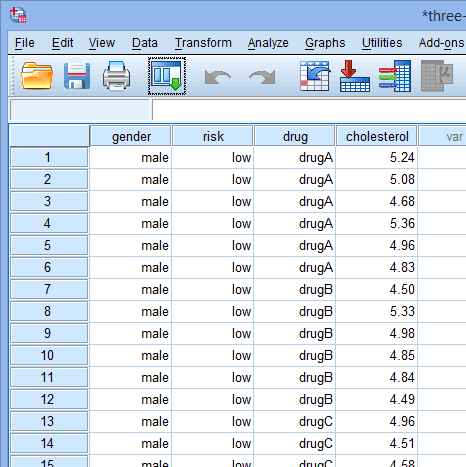Published with written permission from SPSS Statistics, IBM Corporation.

If you are still unsure how to correctly set up your data in SPSS Statistics to carry out a three-way ANOVA, we show you all the required steps in our enhanced three-way ANOVA guide.

## Test Procedure in SPSS Statistics

The six steps below show you how to analyse your data using a three-way ANOVA in SPSS Statistics when the six assumptions in the previous section, Assumptions, have not been violated. At the end of these six steps, we show you how to interpret the results from this test. If you are looking for help to make sure your data meets assumptions #4, #5 and #6, which are required when using a three-way ANOVA and can be tested using SPSS Statistics, you can learn more in our enhanced guides here.

• Click Analyze > General Linear Model > Univariate... on the main menu, as shown below: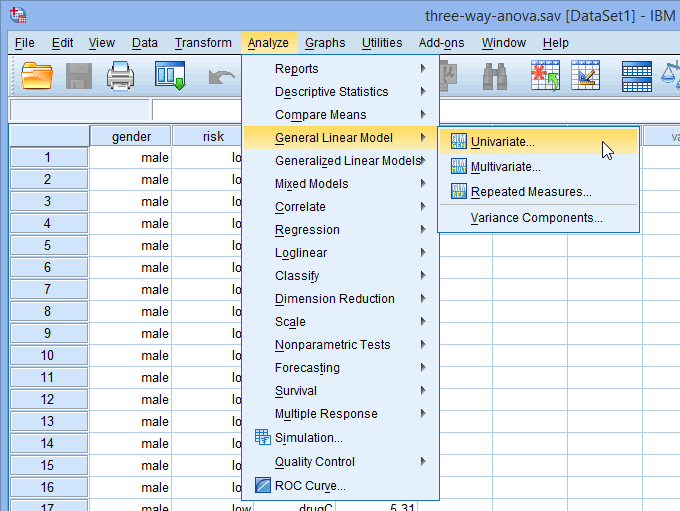Published with written permission from SPSS Statistics, IBM Corporation.

You will be presented with the Univariate dialogue box, as shown below: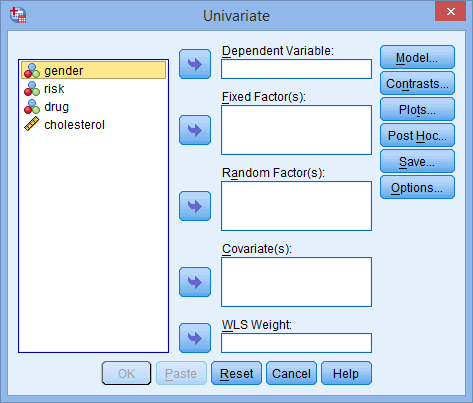Published with written permission from SPSS Statistics, IBM Corporation.

• Transfer the dependent variable, cholesterol, into the Dependent Variable: box and the independent variables – gender, risk and drug – into the Fixed Factor(s): box, using the appropriate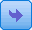buttons. You will end up with a screen similar to the one below: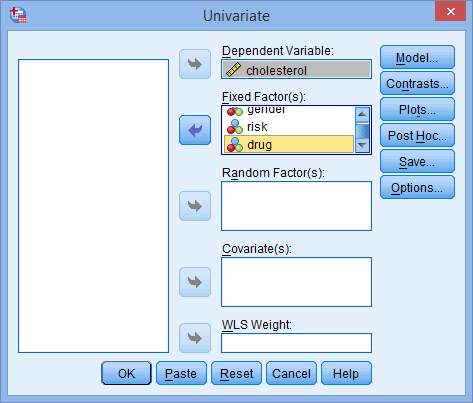Published with written permission from SPSS Statistics, IBM Corporation.

• Click the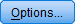button and you will be presented with the Univariate: Options dialogue box, as shown below: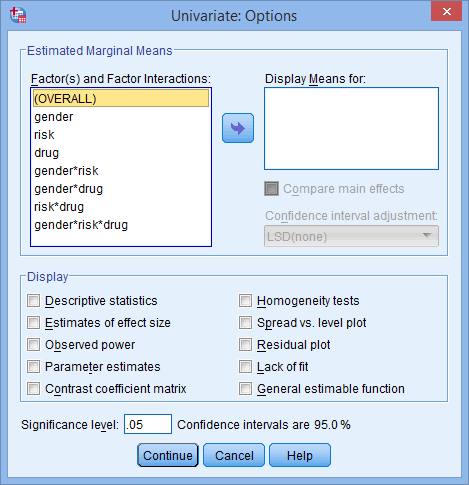Published with written permission from SPSS Statistics, IBM Corporation.

• Transfer the three-way interaction term, gender*risk*drug, from the Factor(s) and Factor Interactions: box to the Display Means For: box by highlighting it and clicking thebutton. Then select the Descriptive statistics and Homogeneity tests options in the –Display– area. You will end up with the screen shown below: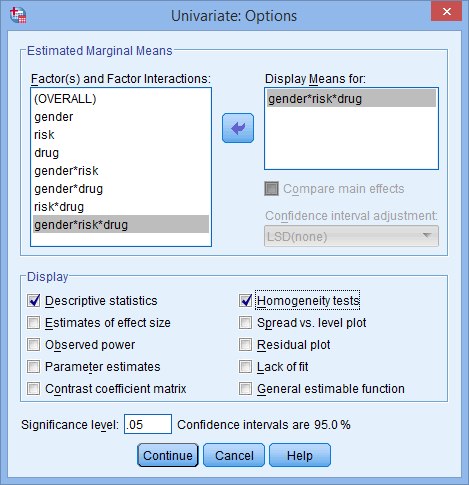Published with written permission from SPSS Statistics, IBM Corporation.

• Click the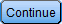button and you will be returned to the Univariate dialogue box.

• Click the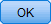button. This will generate the output.

Join the 10,000s of students, academics and professionals who rely on Laerd Statistics.

## Three-way ANOVA result

The primary goal of running a three-way ANOVA is to determine whether there is a three-way interaction between your three independent variables (i.e., a gender*risk*drug interaction). Essentially, a three-way interaction tests whether the simple two-way risk*drug interactions differ between the levels of gender (i.e., differ for "males" and "females").

To determine whether you have a statistically significant three-way gender*risk*drug interaction, you need to consult the "gender * risk * drug" row in the Tests of Between-Subjects Effects table, as highlighted below: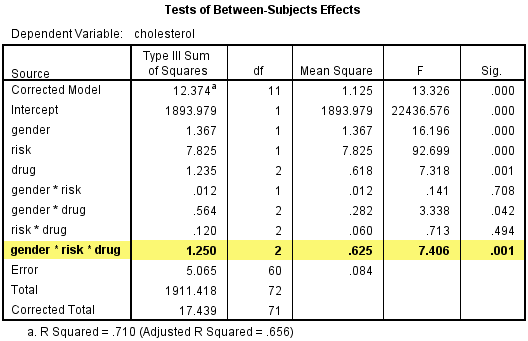Published with written permission from SPSS Statistics, IBM Corporation.

The "Sig." column presents the statistical significance level (i.e., p-value) of the three-way interaction term of the three-way ANOVA. You can see that the statistical significance level of the three-way interaction term is .001 (i.e., p = .001). This value is less than .05 (i.e., it satisfies p < .05), which means that there is a statistically significant three-way gender*risk*drug interaction effect.

You could report this result as follows:

• General

There was a statistically significant three-way interaction between gender, risk and drug, F(2, 60) = 7.406, p = .001.

In our enhanced three-way ANOVA guide (see our Plans & Pricing), in addition to testing all the assumptions, we show you how to follow up a three-way ANOVA result with simple two-way interactions, followed by simple simple main effects and, finally, simple simple comparisons. We show you at every stage how to run these tests, interpret and report them and how to write up all your results. Check out our low prices, which give you access to all our enhanced guides: Plans & Pricing.